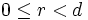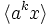# Subgroup structure of dihedral groups

(diff) ← Older revision | Latest revision (diff) | Newer revision → (diff)

We study here the subgroup structure of finite dihedral groups. See subgroup structure of infinite dihedral group for the subgroup structure of the infinite dihedral group.

For any natural number$n$, we define:$D_{2n} := \langle a,x \mid a^n = x^2 = e, xax^{-1} = a^{-1} \rangle$.

For$n \ge 3$,$D_{2n}$ is the group of symmetries in the regular$n$-gon in the plane.

There are two kinds of subgroups:

1. Subgroups of the form$\langle a^d \rangle$, where$d | n$. There is one such subgroup for each$d$. The total number of such subgroups is$\tau(n)$ or$\sigma_0(n)$, i.e., the number of positive divisors of$n$.
2. Subgroups of the form$\langle a^d, a^rx\rangle$ where$d | n$ and$0 \le r < d$. There are thus$d$ such subgroups for each such divisor$d$. The total number of such subgroups is$\sigma(n)$ or$\sigma_1(n)$, i.e., the sum of positive divisors of$n$.

We consider various cases when discussing subgroup structure:

• The special cases$n = 2, n = 4$.
• The special case where$n$ is a power of$2$.
• The special case where$n$ is odd.

## The subgroup$\langle a \rangle$

Further information: Cyclic subgroup of dihedral group

### The special case of$n = 2$

In the case$n = 2$, the group$D_{2n}$ is the Klein four-group:$D_4 = \langle a,x \mid a^2 = x^2 = e, ax = xa \rangle$.

For this, the subgroup$\langle a \rangle$ is a normal subgroup, but not a characteristic subgroup.

### The case$n \ge 3$

In the case$n \ge 3$, the subgroup$\langle a \rangle$ is the unique cyclic subgroup of order$n$. It satisfies the following properties:

### The case$n \ne 4$

In the case$n \ne 4$, the subgroup$\langle a \rangle$ is the centralizer of commutator subgroup, i.e., it is the centralizer in$D_{2n}$ of the commutator subgroup of$D_{2n}$, which is$\langle a^2 \rangle$.

### Odd versus even$n$

When$n$ is odd, the cyclic subgroup of order$n$ in the dihedral group of order$2n$, satisfies the following properties:

None of these properties are satisfied when$n$ is even.

Also, when$n$ is odd, the cyclic subgroup of order$n$ is the commutator subgroup.

## The subgroup$\langle x \rangle$

### General facts

• Its normalizer is$\langle a^{n/2}, x \rangle$ when$n$ is even. When$n$ is odd,$\langle x \rangle$ is self-normalizing.
• The conjugate subgroups to this subgroup are subgroups of the form$\langle a^{2r}x \rangle$. When$n$ is odd, this includes all subgroups of the form$\langle a^k x \rangle$, whereas if$n$ is even, this includes only half the subgroups of the form$\langle a^k x \rangle$. There are thus$n/2$ conjugate subgroups if$n$ is even and$n$ conjugate subgroups if$n$ is odd.
• If$n = 2$ ,there are three automorphic subgroups,$\langle a \rangle, \langle x \rangle, \langle ax \rangle$. If$n \ne 2$, the automorphic subgroups to this subgroup are subgroups of the form$\langle a^r x \rangle$. There are thus$n$ of them if$n \ne 2$.
• The normal closure of$\langle x \rangle$ is$\langle a^2, x \rangle$. This is the whole group if$n$ is odd and is a subgroup of index two if$n$ is even.

### The special case of$n = 2$

When$n = 2$, the dihedral group is the Klein four-group, and$\langle x \rangle$ is a normal subgroup. There is no other$n$ for which$\langle x \rangle$ is a normal subgroup.

### The case$n \ge 3$

Some properties satisfied whenever$n \ge 3$ are:

### Odd versus even$n$

When$n$ is odd, then the subgroup$\langle x \rangle$ satisfies the following properties:

On the other hand, when$n$ is even, this subgroup satisfies none of these properties.

### The case where$n$ is a power of two

When$n$ is a power of two, the subgroup$\langle x \rangle$ satisfies the following properties:

• Subnormal subgroup: In particular, when$n = 2^k$,$\langle x \rangle$ is a$k$-subnormal subgroup.